## Wednesday, January 28, 2009

I have always been confused by naming of commands available for snapshots in VirtualBox. Especially, what is the difference between discard snapshot and discard current snapshot and state. For that reason, I made small experiment. I made new virtual machine (without any snapshots) and I performed the following operations:
2. Take snapshot named a
4. Take snapshot named b
6. Take snapshot named c
7. Remove all dirs and save state of machine
As a result, the my snapshots were as follows:At this point, I had three possibilities:I wanted to see what would happen if I had performed 'discard current snapshot and state' or 'revert to current snapshot'. Remember that in point 7 above, I removed all my folders, therefore Current State (changed) refers to the guest OS without the folders (i.e. without made_before_a, made_before_b and made_before_c).

### Discard current snapshot and state

This option removed snapshot c and the Current State (changed).After starting my guest OA I had only folders made_before_a and made_before_b!

### Revert to current snapshot

After this my guest os was in a state at which snapshot c had been taken (i.e. folders made_before_a, made_before_b and made_before_c were restored)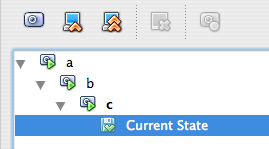Final option is discard snapshot.This option removes the snapshots but not the current state. In other words, I could remove all my snapshots (named a,b, and c) and I was left only with my current state (i.e. no folders)!

### Conclusions

• Revert to current snapshot - it moves guest os to the state it was when the snapshot was made. All changes made since the snapshot creation time will be lost.
• Discard current snapshot and state - it moves guest os to the state of the previous snapshot. Current snapshot and all changes are lost. In my example, performing operation 'discard current snapshot and state' on snapshot c, moved my systems to state of snapshot b.
• Discard snapshot- it removes a snapshot, but not the changes made since the creation of the snapshot.

Hope it will be useful and hope I did not make any mistake (apart from my English :-)).

### Alternative source of info

This topic was previously discussed. Also, VirtualBox documentation might be handy.

## Tuesday, January 27, 2009

### Vim: setting background color

`:highlight Normal ctermbg=black ctermfg=white`Tab size to 4`:set tabstop=4:set ts=4`Specify syntax`set syntax=html`

### Matlab: Working with text data files using Perl

Although, these posts were written a while ago, for Matlab 2007a, they might be still useful.

### Matlab: hash tables

Unfortunately, Matlab does not have hash table functionality by itself. However, Matlab is based on Java, and provides programing interface to java classes. Consequently, it is possible to use Hash tables from Java!

Previously, I described one possible solution to that problem using Java hash tables. Although, it was written a while ago, for Matlab 2007a, it still should be useful.

### Matlab: making Bland and Altman plots

To do the Bland and Altman plot, we have to compute the differences between the instruments and the mean of both instruments for all the paired values.

For instance`A=[749 583 740 235 735 971 867 86 366 369]B=[685 598 789 368 206 87 772 206 388 552]blandAltmanPlot(A,B);`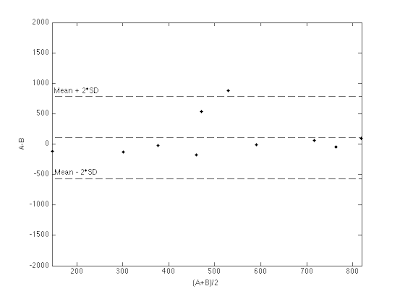`function blandAltmanPlot(A,B)%reference: Y H Chan, Biostatistics 104:%Correlational Analysis,%Singapore Med J 2003 Vol 44(12) : 614-619meanAB=(A+B)./2;difff=A-B;meanDiff=mean(difff);stdDiff=std(difff);meanp2D=meanDiff+2*stdDiff;meanm2D=meanDiff-2*stdDiff;n=length(difff);minD=min(meanAB)-0.1;maxD=max(meanAB)+0.1;figure;plot(meanAB,difff,'.k')hold on;plot([minD; maxD],ones(1,2)*meanp2D,'--k');text(minD+0.01,meanp2D+0.01,'Mean + 2*SD');hold on;plot([minD; maxD],ones(1,2)*meanm2D,'--k');text(minD+0.01,meanm2D+0.01,'Mean - 2*SD');hold on;plot([minD; maxD],ones(1,2)*meanDiff,'--k');xlim([minD maxD]);xlabel('(A+B)/2');ylabel('A-B');`
The excel spreadsheet with the example of Bland and Altman plots is here. These two programs calculated the same statistics.

### Excel: Confidence interval

You can use both t and z distribution to create confidence interval around sample mean. You use t when the sample size is less than 30 (n<30).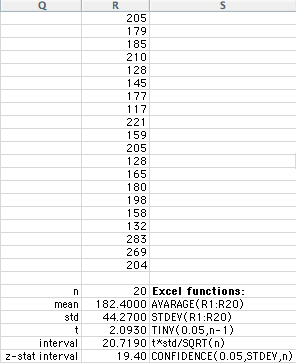Hence with 0.05 (95%) level of confidence our interval is: mean±interval i.e. 182.4±20.719 and 45±4.6924, respectively.
For illustration only there is also z statistic interval shown (19.4 and 4.39 respectively). As can be seen, using z instead of t makes the interval to be smaller.

If one wants to use for example, 0.01 (99%) level of confidence one must use TINV(0.01,n-1) for t distribution and CONFIDENCE(0.01,STDEV,n) for z distribution.
In our case if we use 0.01 we get interval for t distribution equal to 28.3207 for the first example and 6.4141 for the second example. Interval for z is 25.50 and 5.77 respectively.

Briefly:`Interval using z distribution is narrowerthan for t distribution`
The same but in R:`>X1 <- scan()1: 205 179 185 210 128 145 177 117 221 159 205 128 165 180 198 158 132 283 269 204> a <- mean(X1)> s <- sd(X1)> n <- length(X1)> errZ <- qnorm(0.975)*s/sqrt(n)> errT <- qt(0.975,df=n-1)*s/sqrt(n)`

### Excel: Paired t-test

I always forget what must be the value of P must be to reject or not to reject the null hypothesis in paired t-test. So lets explain by the example.`H0 - null hypothesis - there is nosignificant differencebetween method A and BH1 - alternative hypothesis - there is difference(two tail test)`For example [with 5% (a=0.05) level of significance ]: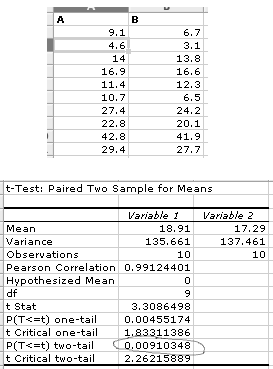Based on the above results I can say that: "since P=0.009103483 and this is lover than a=0.05 (P<0.05), I can claim that":`I'm 95% (a=0.05) sure that there is significantdifference between A and B, because (P<0.05).`On the other hand, we can have: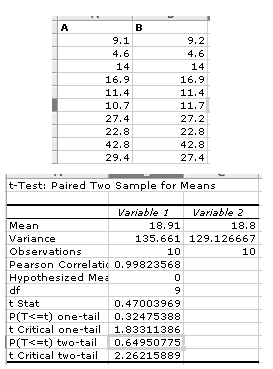In this case P=0.649507752, and I can claim that:`I'm 95% (a=0.05) sure that there is no significantdifference between A and B, because (P>0.05).`Above paired t-test was performed in Excel.

### Matlab: Coefficient of correlation and determination

Both coefficients are used to measure the relationship between two variables. For example lets assume that we want to check whether there is a correlation between the size of the store (in thousands of square feet) (X variable) and annual sales (in million dollars) (Y variable):

X=[1.7 1.6 2.8 5.6 1.3 2.2 1.3 1.1 3.2 1.5 5.2 4.6 5.8 3.0]
Y=[3.7 3.9 6.7 9.5 3.4 5.6 3.7 2.7 5.5 2.9 10.7 7.6 11.8 4.1]

Using Matlab/Octave we can calculate Coefficient of correlation (r) and Coefficient of determination (r2) in a following way:
`c=corrcoef([X' Y']);r=c(1,2);r2=r^2;`
In our example we get r=0.95088 and r2=0.90418.
Now what does it mean? Coefficient of determination measures the proportion of variation in Y that is explained by the X. In other words, we can say that 90.4% of the change in Y can be explained by the change in X. In our case we can conclude that 90.4% of change in annual sales is explained by the change in store size. The rest (9.6%) depends on the other factors like localization, staff, management, etc.

Bellow scatter plot of X and Y:The above scatter plot can be generated in Matlab/Octave by:
`figure;plot(X,Y,'+r');title('Scatter plot');`

## Monday, January 26, 2009

### VirtualBox: port forwarding

My host: windows XP
My guest: ubuntu 8.04.1

To redirect all connections from port 80 (www server) of the host machine to port 80 of theguest operating system being I used the following commands in windows xp console: `VBoxManage setextradata "ubuntu-server" "VBoxInternal/Devices/pcnet/0/LUN#0/Config/Apache/Protocol" TCPVBoxManage setextradata "ubuntu-server" "VBoxInternal/Devices/pcnet/0/LUN#0/Config/Apache/GuestPort" 80VBoxManage setextradata "ubuntu-server" "VBoxInternal/Devices/pcnet/0/LUN#0/Config/Apache/HostPort" 80`
"Apache" is an arbitrary name, and "ubuntu-server" is the name of my virtual machine with Ubuntu.

Ports can be redirected only when VirtualBox is turned off.

### Matlab: Compile m file in Matlab 7.4 on Mac X

I tried to compile one simple m file - get_pad_load.m (see previous post).
First, when I tried to use mcc -m test.m I got error `dyld: Library not loaded: ../../bin/maci/libmwcompiler.dylib Referenced from: /Applications/MATLAB74/bin/maci/mcc Reason: image not foundTrace/BPT trap`

To repair it I indicated paths to missing libraries ` export DYLD_LIBRARY_PATH="/Applications/MATLAB74/bin/maci:/Applications/MATLAB74/sys/os/maci"`
This worked. I was able to compile my file, and run it from console as an executable.

### sed: some substitute text examples

Substitute text1 with text2 in file /etc/apt/sources.list:
`cat /etc/apt/sources.list | sed 's/text1/text2/g' > out.txt `
or with different 'slash':`cat /etc/apt/sources.list | sed 's|text1|text2|g' > out.txt `
Multiple substitution: `cat /etc/apt/sources.list | sed -e 's/text1/text2/g' -e 's/text3/text4/g' > out.txt `

Delete lines containing 'blabla' string:`cat some.txt | sed -e '/blabla/d' > out.txt`

## Sunday, January 25, 2009

### Matlab: Simple linear regression analysis

Simple linear regression analysis is used to predict the values of one variable (dependent variable - X) based on the values of one other variable (independent variable - Y). In simple linear regression analysis, relationship between two variables is represented by the straight line (prediction line) fitted (Y = aX + b) to the scatter plot of those variables.

First, lets start by defining our working example. Lets assume that we want to examine relationship between the store size (in thousands of square feet) (X variable) and its annual sales (in million of dollars) (Y variable). We have sample of 14 stores, and data about them:
X= [1.7 1.6 2.8 5.6 1.3 2.2 1.3 1.1 3.2 1.5 5.2 4.6 5.8 3.0];
Y= [3.7 3.9 6.7 9.5 3.4 5.6 3.7 2.7 5.5 2.9 10.7 7.6 11.8 4.1];

Determining prediction line (Y=aX+b)

Prediction line (Y = aX + b) where: a is slope of the fitted line, and b is intercept of the line with Y axis. To determine those parameters we can use Matlab/Octave code:
`p=polyfit(X,Y,1);a=p(1); %slopb=p(2); %icept`
In our case a=1.67 and b=0.9645; hence the line is: Y = 1.67X + 0.9645.
What does it mean? The slop means that for each increase of 1 unit in X, the mean value of Y is estimated to increase by 1.67 units. In our case it means that if size of store increases by 1 thousands square feet, the mean annual sales is estimated to increase by 1.67 millions of dollars. On the other hand, the intercept represents the mean value of Y when X equals 0. In our case intercept has no practical meaning because size of store cannot be 0.

Scatter diagram and fitted regression line are show below:Standard error of the estimate - Syx

Prediction using simple regression line is not perfect; hence, statistic that measure the variability of the actual Y values from the predicted Y values must be developed, in the same way as standard deviation was developed to measure the variability of value around the mean. The standard deviation around the prediction line is called standard error of the estimate - Syx.
Calculation of Syx in Matlab/Octave is:
`x=X';y=Y'; %from row vector to column vector.Sxx=sum((x-mean(x)).^2);Syy=sum((y-mean(y)).^2);Sxy=sum((x-mean(x)).*(y-mean(y)));SSE=Syy-a*Sxy;S2yx=SSE/(n-2);%Standard error of estimate:Syx=sqrt(SSE/(n-2));`
In our case we get Syx = 0.9664. The standard error of the estimate is measured in the same units as the dependent variable (Y). In our case defendant variable Y is Annual sales, and our standard error of the estimate is equal to 0.9664 millions of dollars. We can express this value on the scatter plot as below: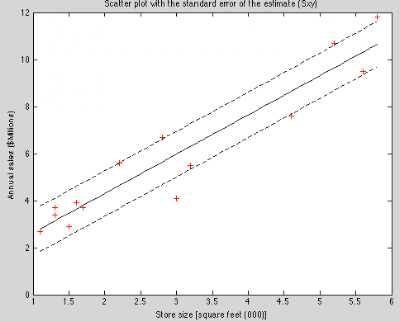Test whether there is a significant linear relationship

In this section we want to test if there exist a significant (with confidence of 95%; hence, alpha=0.05) linear relationship between X and Y. To do this we can perform t-test for the slop. We do this by stating null and alternative hypothesis as fallows:`H0 : A = 0 (There is no linear relationship)`
`H1 : A = 0 (There is a linear relationship)` where A is the slop of the relationship in the population of X and Y. I remained that we have only sample of size 14 from the population. We perform regression analysis on the sample trying to infer relationship in the populations X and Y.
The t statistics equals: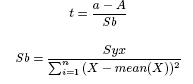.
We calculate t and P-value and check whether P-value>alpha=0.05; hence, whether we can reject or not our null hypothesis.
`%we use date from the earlier code aboveSb=sqrt(S2yx/Sxx);A=0;t=(a-A)/Sb;Pvalue=2*(1-tcdf(abs(t),n-2));% in older Octave (2.1) use t_cdf!!!` hence t=10.64 and P-value=1.8227e-07 lower than alpha=0.05 so we reject null hypothesis and conclude that for 95% there is significant linear relationship between mean annual sales and the size of the store.

The confidence interval estimate

Regression analysis can be used to predict values of Y based on X. In our case we want to be able to predict the annual sales based on the size of the store. The simplest way to do it, just just point estimate using our line equation Yy = a*Xx + b = 1.67Xx + 0.9645, where Yy is our prediction and Xx is the value of X that we make prediction for. For example, if one asks what is estimated mean annual sales for the store of size 4000 square feet ( Xx=4.0), we calculate
Yy = 1.67*4.0 + 0.9645 = 7.644
Hence, the predicted mean annual sales for the store of size of 4000 square fit
is 7.644 million dollars.
This kind of prediction is called point rediction, and we know that our regression line is not perfect due to standard error of the estimate (Syx). We would prefer to be more statistically correct; hence, we should create confidence interval for our predictions.
Confidence interval estimate for the mean of Y: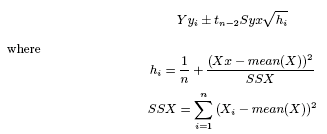`Xx=4.0; %predict Y for X=4.Yy=b+a.*(Xx); %Y predicted from regression line.SSX=sum((X-mean(X)).^2);h=1/n + ((Xx-mean(X)).^2)/SSX;tn2= tinv(1-alpha/2,n-2); %tn-2 - t criticalinterval=tn2.*Syx.*sqrt(h);` hence the interval is 0.673.
For example if we want to know the confidence interval of the mean annual sales for the store of size 4.000 square feet ( Xx=4.0), after calculations we get: 7.644 ± 0.673. In other words the mean annual sales are between 6.971 and 8.317 (million of
dollars) for the population of stores with 4000 square feet. If we calculate interval for all the X values in our sample, we can show this interval graphically:For now that is everything what I have to say about this topic; however, this topic is much more longer and complex. But for most situations, it is a good start.

### Matlab: Creating sinusoidal surfaces

2-D sinusoidal surfaces are used in image processing e.g. to evaluate implementation of Gabor filters, Wavelet and Fourier transformations, fractal analysis, etc.. Also they are very useful when one tries to explain how the above operation works, since all of the above are based on trigonometric functions (apart from fractals).

2-D sinusoidal surface is just an sinus function in 2D domain. On the other words, it is image of two-dimensional function sin.`function I=sinSurf(varargin)%create sinusoidal 2D function (image)%INPUT% - theta - angle in radians (default pi/2)% - lambda - frequency (default 0.5)% - Isize - output image size (default 256);% - delta - phase (default 0.0);%OUTPUT% - I - double matrix double (-1..1) of sinusoidal functiontheta=pi/2;Isize = 256;mag=0.5;lambda=0.5;delta=0;if nargin >= 1theta=varargin{1};endif nargin>=2lambda=varargin{2};endif nargin>=3Isize=varargin{3};endif nargin>=4delta=varargin{4};endI=zeros(Isize,Isize);cosa=cos(theta);sina=sin(theta);for x=1:Isizefor y=1:Isizexprime = (x*cosa+y*sina)/Isize;valu = mag*sin(2*pi*(xprime/lambda)-delta);I(x,y)=valu;endend`

Below some examples:

a) For: theta=pi/2;`s=sinSurf();imshow(s,[-1 1]);colormap(jet);colorbar;figure, mesh(s); axis([1 256 1 256 -1 1]); axis square;`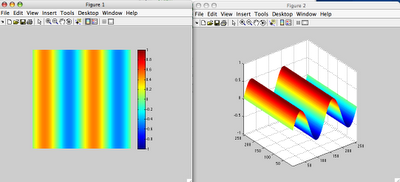b) For: theta=pi/4;`s=sinSurf(pi/4);imshow(s,[-1 1]);colormap(jet);colorbar;figure, mesh(s); axis([1 256 1 256 -1 1]); axis square;`c) For: theta=pi/2; lambda=0.25;`s=sinSurf(pi/2,0.25);imshow(s,[-1 1]);colormap(jet);colorbar;figure, mesh(s); axis([1 256 1 256 -1 1]); axis square;`### bash: using find plus grep command

The problem is to scan all files of some name (.e.g. *.css) and to find only those that contain specific string (e.g. 702px). ` find ./ -name "*.css" -exec grep '702px' {} \; -print`
The command finds all .css files that contain string "702px".

### Linux: Making backup using tar command

tar file
Make a tar file:`tar cvf mybackup.tar some_folder/`
List a tar file:`tar tvf mybackup.tar`
Unpack tar file:`tar xvf mybackup.tar`
tar.gz file
Make a tar.gz file:`tar czvf mybackup.tar.gz some_folder/`
Unpack tar.gz file:`tar xzvf mybackup.tar.gz`
List a tar.gz file:`tar tzvf mybackup.tar`
excluding some files
`tar cvf mybackup.tar some_folder/ --exclude=*.mp3 --exclude=*.avi`
example
`tar cvf /Volumes/LaCie/staff_from_before_2008_05_20/from_mac/Desktop.tar Desktop/ --exclude=*.avi --exclude=*.rmvb --exclude=*.mp3 --exclude=*.jpg --exclude=*.mat`

The post was moved here.

Just be very careful! For me, changing the name of the blogspot address was not very good idea!. The current address of this blog (shortrecipes.blogspot.com) is a new name, created about 1.5 months ago. This blog exists for about three years, and during this time I have written over 120 posts. Unfortunately, once I changed the name of this blog to shortrecipes.blogspot.com, Google removed or lost indexes to all the posts that had been written when the blog had been known under old name. Currently, Google indexes only posts that have been created since the time blogspot address was changed (i.e. about 1.5 months ago).Therefore, if one wants to changes its blogspot address, one should be very careful. It is possible that similar situation may happen.

### Temporary solution

found out that to make Google to index some of my old posts, I have to recreate them. In another words, I take a post created e.g. 1 year ago, and I need to copy and paste its contents to the new, empty post. This is not the perfect solution, but I can make the most popular posts visible in Google again.

### Python: Example of Threads and Queue

Just an example of making thread workers, that executes tasks from the task queue. I used it to check if a PC box with multicore processor is really 100% busy with threads. The code multiplies some large, random matrices, so it does not do anything useful. However, it can serve as an example or a template of a simple multithread application.
`import threading, Queue, timefrom scipy import *class MultiplyMatrixes(threading.Thread): def __init__(self,queue,name): self.queue=queue threading.Thread.__init__(self,name=name) def run(self): while True: #wait until there is a task # in queue. Get task from gueue. n=self.queue.get() print "Spawning: ",self.getName(),n if n !=None: # do the task p=self.doMultiplication(n) print "Finished:", self.getName(),n,p #indicate that the task has been #complited self.queue.task_done() def doMultiplication(self,n): m1=matrix(rand(n,n)) #some random matrix m2=matrix(rand(n,n)) #some random matrix m3=m1*m2 return m3.mean() # return meanclass MainThread(): def __init__(self,noOfThreads): self.no=noOfThreads def doTest(self): t1=time.time() self.test() print "Elapsed time",time.time()-t1 def test(self): #change maxMtxSize for longer or shorter # execution minMtxSize,maxMtxSize=100,1200 nT=range(minMtxSize,maxMtxSize,100) #make task queue queue=Queue.Queue() #create number of workers (threads) for i in range(self.no): p=MultiplyMatrixes(queue,name=str(i+1)) p.setDaemon(True) p.start() #put some tasks into the queue for n in nT: queue.put(n) #wait until the queue is finshed (no more tasks) queue.join() #give some time for threads #to finished before exiting. time.sleep(1) #Otherwise the follwing error is more likely to occur: # Exception in thread 1 (most likely raised during interpreter shutdown): # Traceback (most recent call last): # File "/usr/lib/python2.5/threading.py", line 486, in __bootstrap_inner # File "multithreads.py", line 19, in run # File "/usr/lib/python2.5/Queue.py", line 165, in get # File "/usr/lib/python2.5/threading.py", line 209, in wait # : 'NoneType' object is not callable print "Finished!!!" def test(): MP=MainThread(noOfThreads=4) MP.doTest() if __name__ == '__main__': test()`Example result:`Spawning: 1 100Finished: 1 100 25.3551569392Spawning: 2 200Spawning: 3 300Spawning: 1 400Spawning: 4 500Finished: 2 200 49.9585540799Spawning: 2 600Finished: 3 300 75.163025977Spawning: 3 700Finished: 1 400 99.7075107712Spawning: 1 800Finished: 4 500 124.849660963Spawning: 4 900Finished: 2 600 149.99426813Spawning: 2 1000Finished: 3 700 175.035573614Spawning: 3 1100Finished: 1 800 199.893719979Finished: 4 900 225.168134578Finished: 2 1000 250.157828058Finished: 3 1100 275.025345136Finished!!!Elapsed time 73.96342206`

### Python: Python Imaging Library - 16 bit images

The problem described in the previews post about 16-bit images in python can be overcome by converting image into numpy array, operating in numpy array, and finally converting array into image and saving it:
`def flip_horizontally2(inDir,inFile,outFile=None):''' Open 16-bit or 8-bit image, flop it horizontally,and save it.'''imgpath=inDir+inFileim = Image.open(imgpath)print im.mode,im.size, im.size#convert image into array according to im.modeif im.mode in ("L",): a = fromstring(im.tostring(), uint8)elif im.mode in ("I;16",): a = fromstring(im.tostring(), uint16)a.shape = im.size, im.sizea=fliplr(a) #flip horizontally#convert array into image according to a.dtypeif a.dtype == uint8: mode = "L"elif a.dtype == uint16: mode = "I;16"else: raise ValueError, "unsupported image mode"out=Image.fromstring(mode, (a.shape, a.shape), a.tostring())#save imageif outFile is None: outFile=inFilebase=os.path.splitext(outFile)out.save(inDir+base+'_flopped2.tiff')`

Using this function instead of the one used before produces correct results.

### imagemagick: crop all image files

The code below crops all tif images to 61x61 pixels measured from upper left corner of an image (+0+0) and converts and image to 8-bit gray-scale levels.
` for f in *.tif; do convert -colorspace Gray -depth 8 +gravity -crop 61x61+0+0 +repage \$f ../california2/\$f; done`

### Matlab: Compiling m file on Mac X

I tried to compile one simple m file - get_pad_load.m (see previous post).
First, when I tried to use mcc -m test.m I got error `dyld: Library not loaded: ../../bin/maci/libmwcompiler.dylib Referenced from: /Applications/MATLAB74/bin/maci/mcc Reason: image not foundTrace/BPT trap`

To repair it I indicated paths to missing libraries ` export DYLD_LIBRARY_PATH="/Applications/MATLAB74/bin/maci:/Applications/MATLAB74/sys/os/maci"`
This worked. I was able to compile my file, and run it from console as an executable.

### Python: an image to an array and an array to an image

Converting 8-bit gray-scale level images to numpy array, and generating a 8-bit gray-scale level image from array.

### Python: check if a string is a number

To check if a string represents a number, this may be used:`def isnum(s): try: float(s) except ValueError: return Falsereturn True`
Examples:
`In : isnum('abs')Out: FalseIn : isnum('2.5')Out: TrueIn : isnum('-2.5')Out: TrueIn : isnum("2e-3")Out: TrueIn : isnum("2e-3 a")Out: FalseIn : isnum("2e-3 ")Out: TrueIn : isnum(" 1.1e+3 ")Out: TrueIn : isnum(" 52 ")Out: True`

### Mac X: Port forwarding from Host OS to guest OS in VirtualBox 2.0.2

I run ubuntu-server on my VirtualBox 2.0.2. In order to run ubuntu-server on my VirtualBox I followed this site. The reason is that Ubuntu-server uses PAE (physical address extension) which is not supported in VB (this feature is still in beta in VB). Once this has been fixed, I was able to connect to web server running within VB from my Mac OS. For this I used the following commands in Mac X:
`VBoxManage setextradata "ubuntu-server" "VBoxInternal/Devices/pcnet/0/LUN#0/Config/Apache/Protocol" TCPVBoxManage setextradata "ubuntu-server" "VBoxInternal/Devices/pcnet/0/LUN#0/Config/Apache/GuestPort" 80VBoxManage setextradata "ubuntu-server" "VBoxInternal/Devices/pcnet/0/LUN#0/Config/Apache/HostPort" 8080`To remove this forwarding just execute the same commands without 80,8080 and TCP.

The same should work in windows xp. Command netstat -a shows connections.

When porting VB ubuntu-server from Mac X to Windows XP, there was a problem with VB - no internet. I run dhclient and change entry in /etc/network/interface. After that internet in VB was working, and web server also.

## Wednesday, January 21, 2009

### oMedia cms: Potential SQL inject vulnerability

oMedia is a Polish cms for shearing multimedia files. During some modifications of this cms, I found that there might be possible SQL injection vulnerability. Specifically, the problem is with the search panel. For example, performing a search for a file using a string that contains single quote (') results in the MySQL error:The error shows full MySQL command! This might be a potential for SQL injection attacks on the websites using this cms. Please don't panic! I'm not saying that there is vulnerability, I'm just pointing out that there might be vulnerability.
The solution to this problem is quite simple. In a file searchFile.php the following code `foreach (\$keywords as \$keyword) {if (\$i > 0) {\$filter .= ' OR ';}\$filter .= "f.name LIKE '%\$keyword%' OR f.description LIKE '%\$keyword%' ";\$i++;}` should be changed to this:`foreach (\$keywords as \$keyword) {\$keyw=addslashes(\$keyword);if (\$i > 0) {\$filter .= ' OR ';}\$filter .= "f.name LIKE '%\$keyw%' OR f.description LIKE '%\$keyw%' ";\$i++;}`The above solution simply adds addslashes() function. Hope this works.

## Tuesday, January 13, 2009

### Prado framework: problems running demo applications

PRADO is a component-based and event-driven programming framework for developing web applications in PHP5. Beginners wanting to learn Prado may have problems when they want to run demo applications on their own server. Some demos simply don't work. Particular, I had problems on xampp 1.7 for Windows with AJAX Chat, address-book and northwind-db demos. The problem is that they use sqlite3 database and they require pdo_sqlite and php_pdo_sqlite PHP extensions. However, the PHP in xampp 1.7 for Windows(PHP 5.2.8) a comes with these extensions for only sqlite2, not sqlite3!. For that reason when I tried to run e.g. AJAX Chat demo I got the "DbConnection failed to establish DB connection: could not find drive" error:The solution to this problem was, at least for me, to convert sqlite3 databases of these demos to sqlite2.

### AJAX Chat demo

The online example of this demo is here. As previously indicated, when I wanted to use the demo I was greeted with DbConnection error. This indicates that there is some problem with database connections. AJAX Chat demo requires pdo_sqlite extensions. So first thing that I did was to check if my PHP has such extensions. Before proceeding I made sure that these extensions were enabled in php.ini or php5.ini. To check if I have these extensions I used `<?PHP phpinfo();?>`. These resulted, among other things, with the following:This cleary shows that my pdo_sqlite driver is sqlite2. For that reason I converted the chat.db database of the AJAX Chat demo from sqlite3 to sqlite2 as follows:`sqlite3 chat.db .dump | sqlite chat2.db`Next, in application.xml I made the following change fromtoFollowing these changes the AJAX Chat demo finally worked:Online demo is here. The problem with this demo was the same as with the AJAX Chat demo, i.e. sqlite database. Since the demo uses Flex the error message was little different: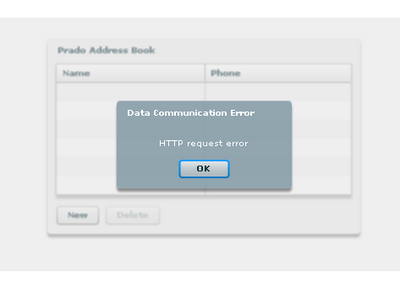To make it work, I converted the demo's sqlite3 database to sqlite2 database as follows:`sqlite3 sqlite.db .dump | sqlite sqlite2.db`. Next application.xml was modified as before and the demo worked: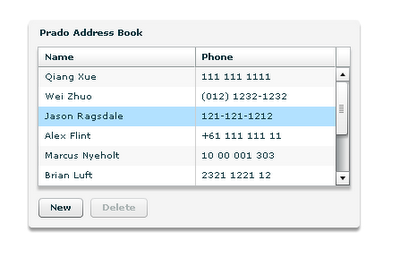### northwind-db demo

The problem with this demo is the same as before. However, this time converting sqlite3 database to sqlite2 database resulted in errors. In another worlds, I could not convert the demo's database. The reason was that the demo's database is much more complicated than that of address-book and chat demos, and sqlite2 returned syntex errors when dumping. At the moment I'm still analyzing AJAX Chat demo, so northwind-db demo is far ahead. When and if I get to it, I try to find some solution this problem.

The two sqlite database created are here.

## Sunday, January 11, 2009

### oMedia cms: adding suggestions to the search panel

oMedia is a Polish cms that is used for websites that provide image and movie files (something ala YouTube). Although it has many useful functions, performing a search for a file on the websites using the cms is not straight forward. The reason is that the search input filed does not provide suggestion while the users writes the query. Hence, I looked into the code of the cms and I modified it. This modifications required changing two files: searchFile.page and searchFile.php. Since oMedia is build on top of Prado framework, it was necessary to change TTextBox component in searchFile.page into TAutoComplete component and modify corresponding searchFile.php.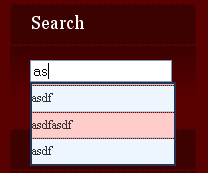The modified two files can be downloaded from here. Therefore, to use the files it is necessary to substitute the two files on one's server (there are located in protected/panels/). Of course be sure to make copy of the original files before substitution. Additionally if the original files had already been modified, the two files must be modified manually.

## Monday, January 05, 2009

### JavaScript: Escape text with HTML entries

When I post some code snippets into this website, often there is a problem with html tags or symbols present in the code. The most common examples are greater and lower symbols: <,>. For that reason I wrote simple html form and some JavaScript that escapes html tags or symbols.

## Input text:

Remove double End of Line characters